PEAK-Rules/Syntax-Matching UserPreferences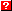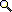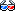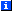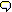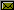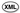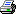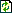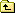The following 283 words could not be found in the dictionary of 50 words (including 50 LocalSpellingWords) and are highlighted below:
Add   And   Bind   Builder   By   Call   Compare   Comparison   Compiling   Const   Contents   Criteria   Each   Error   Except   Expr   Finally   For   Getitem   Identity   If   In   Instance   Is   It   Local   Match   Matching   Non   None   Object   Of   Parsing   Patterns   Predicates   Pseudo   Python   Rules   Signature   Structures   Syntax   Table   Test   The   This   Thus   Traceback   Tree   True   Truth   Ts   Value   Variables   Will   above   actually   adding   against   all   allowing   allows   already   also   always   an   and   any   application   arbitrary   are   argument   around   as   assembled   assembler   atop   back   backquoted   backquotes   based   be   being   between   bind   binding   bindings   binds   bit   build   builder   builtins   but   by   call   can   care   cause   child   children   class   code   codegen   combine   compare   comparing   comparison   comparisons   complex   contents   course   create   created   criteria   data   default   define   defined   determine   dictionary   do   don   each   entry   equal   equality   essentially   exact   example   exception   expr   expression   expressions   extending   for   form   found   from   function   get   globals   hand   identifiers   if   import   in   indentifier   indicating   inserting   intended   intersected   into   is   isn   istype   it   kinds   last   later   len   length   like   lists   locals   location   machinery   mainly   mapping   match   matched   matching   modified   module   more   most   name   named   names   never   new   node   nodes   not   object   of   old   on   once   only   operation   operator   order   other   parameterized   parse   parses   part   pattern   patterns   pe   peak   perform   performing   place   placeholders   predicate   predicates   produces   re   really   recent   recognize   recursive   recursively   refer   rejects   replace   represented   respects   return   returned   right   rule   rules   same   see   sequences   should   show   side   single   snippets   so   special   specified   stored   string   structure   subexpression   such   suitable   syntax   target   test   than   that   the   their   them   themselves   there   they   this   to   tools   treated   tree   true   two   type   types   unchanged   under   underscore   updated   use   used   util   v1   v2   value   values   variable   variables   various   via   we   what   where   which   will   with   within   you

# Matching Python Syntax

The peak.rules.syntax module allows you to define pattern-matching predicates against snippets of parameterized Python code, such that a rule expression like:

```syntax.match(expr, type(`x`) is `y`) and y in Const
```

Will return true if expr is a PEAK-Rules AST of the form:

```Compare(Call(Const(type), (v1,)), ('is', Const(v2)))
```

(where v1 and v2 are arbitrary values).

# Bind Variables

Bind variables are placeholders in a pattern that "bind" themselves to the value found in that location in the matched data structure. Thus, in the example above, `x` and `y` are bind variables, and cause "y" in the later part of the expression to refer to the right-hand side of the is operator being matched. (The arbitrary value v2 in the example above.)

Bind variables are represented within a tree as an AST node created from the variable name:

```>>> from peak.rules.syntax import Bind

>>> Bind('x')
Bind('x')
```

# Compiling Tree-Match Predicates

The match_predicate(pattern, expr, binds) function is used to combine a pattern AST and an expression AST to create a PEAK-Rules predicate object that will match the specified pattern. The binds argument is a dictionary mapping from bind-variable names to lists of expression ASTs, and is modified in-place as the predicate is assembled:

```>>> from peak.rules.syntax import match_predicate
```

Rules defined for this function will determine what to do based on the type of pattern. If pattern is a bind variable, the binds dictionary is updated in-place, inserting expr under the bind variable's name, and True is returned, indicating that this part of the pattern will always match:

```>>> from peak.util.assembler import Local
>>> b = {}

>>> match_predicate(Bind('x'), Local('y'), b)
True

>>> b
{'x': [Local('y')]}
```

If there's already an entry for that variable in the binds dictionary, a more complex predicate is returned, performing an equality comparison between the new binding and the old binding of the variable, and the value in binds is updated:

```>>> match_predicate(Bind('x'), Local('z'), b)
Test(Truth(Compare(Local('z'), (('==', Local('y')),))), True)
```

This is so that patterns like "x is not x" will actually compare the two "x"s and see if they're equal. Of course, if you bind the same variable more than once to equal expression ASTs, you will not get back a comparison, and the binds will be unchanged:

```>>> match_predicate(Bind('x'), Local('z'), b)
True

>>> b
{'x': [Local('y'), Local('z')]}
```

Finally, there is a special exception for bind variables named `_`: that is, a single underscore. Bind variables of this name are never stored in the binds, and always return True as a predicate, allowing you to use them as "don't care" placeholders:

```>>> any = Bind('_')
>>> match_predicate(any, Local('q'), b)
True

>>> b
{'x': [Local('y'), Local('z')]}
```

## Matching Structures and AST nodes

For most node types other than Bind, the predicates are a bit more complex. By default, the predicate should be an exact (istype) match of the node type, intersected with a recursive application of match_predicate() to each of the target node's children. For example:

```>>> b = {}
>>> from peak.util.assembler import *
>>> from peak.rules.codegen import *

>>> b
{}
```

Each child is defined via a Getitem() operation on the target node, so that any placeholders and criteria will target the right part of the tree:

```>>> match_predicate(Add(Bind('x'), Bind('y')), Local('q'), b)

>>> b
{'y': [Getitem(Local('q'), Const(2))],
'x': [Getitem(Local('q'), Const(1))]}
```

Non-node patterns are treated as equality comparisons:

```>>> b = {}
>>> match_predicate(42, Local('q'), b)
Test(Comparison(Local('q')), Value(42, True))

>>> b
{}
```

Except for None, which produces an is None test:

```>>> match_predicate(None, Local('q'), b)
Test(Identity(Local('q')), IsObject(None, True))

>>> b
{}
```

And sequences are matched by comparing their length:

```>>> match_predicate((), Local('q'), b)
Test(Comparison(Call(Const(<... len>), (Local('q'),),...)), Value(0, True))

>>> match_predicate([], Local('q'), b)
Test(Comparison(Call(Const(<... len>), (Local('q'),),...)), Value(0, True))

>>> b
{}
```

And recursively matching their contents:

```>>> match_predicate((Bind('x'), Add(Bind('y'), any)), Local('q'), b)
Signature([Test(Comparison(Call(Const(<... len>), (Local('q'),),...)),
Value(2, True)),
Test(IsInstance(Getitem(Local('q'), Const(1))),

>>> b
{'y': [Getitem(Getitem(Local('q'), Const(1)), Const(1))],
'x': [Getitem(Local('q'), Const(0))]}
```

# Parsing Syntax Patterns

The syntax.SyntaxBuilder class is used to parse Python expressions into AST patterns suitable for use with match_predicate:

```>>> from peak.rules.syntax import SyntaxBuilder, match
>>> builder = SyntaxBuilder({}, locals(), globals(), __builtins__)
>>> pe = builder.parse
```

It parses backquoted identifiers into Bind nodes:

```>>> pe('type(`x`) is `y`')
Compare(Call(Const(<type 'type'>), (Bind('x'),), (), (), (), True),
(('is', Bind('y')),))
```

And rejects all other use of backquotes:

```>>> pe('`type(x)`')
Traceback (most recent call last):
...
SyntaxError: backquotes may only be used around an indentifier
```

In all other respects, it's essentially the same as codegen.ExprBuilder.

## The match() Pseudo-function

This isn't really a function, but you can use it in a predicate string in order to perform a pattern match on a PEAK-Rules AST. It's mainly intended for use in extending PEAK-Rules to recognize and replace various kinds of subexpression patterns (e.g. by adding rules to predicates.expressionSignature()), but it can of course also be used in any other tools you build atop PEAK-Rules' expression machinery.

In this example, we show it being used to define a rule that will recognize expressions of the form "type(x) is y", where x and y are arbitrary expressions:

```>>> from peak.rules.syntax import match

>>> from peak.rules.predicates import CriteriaBuilder
>>> builder = CriteriaBuilder(
...     {'expr':Local('expr')}, locals(), globals(), __builtins__
... )
>>> pe = builder.parse

>>> pe('match(expr, type(`x`) is `y`)')
Signature([Test(IsInstance(Local('expr')),
istype(<class 'peak.util.assembler.Compare'>, True)),
Test(IsInstance(Getitem(Local('expr'), Const(1))),
istype(<class 'peak.util.assembler.Call'>, True)),
Test(Comparison(Getitem(Getitem(Local('expr'), Const(1)),
Const(1))),
Value(Const(<type 'type'>), True)),
Test(Comparison(Call(Const(<... len>),
(Getitem(Getitem(Local('expr'),
Const(1)), Const(2)),), (),
(), (), True)),
Value(1, True)),
Test(Comparison(Call(Const(<... len>),
(Getitem(Getitem(Local('expr'), Const(1)),
Const(3)),), (), (), (), True)),
Value(0, True)),
Test(Comparison(Call(Const(<... len>),
(Getitem(Getitem(Local('expr'), Const(1)),
Const(4)),), (), (), (), True)),
Value(0, True)),
Test(Comparison(Call(Const(<... len>),
(Getitem(Getitem(Local('expr'), Const(1)),
Const(5)),), (), (), (), True)),
Value(0, True)),
Test(Comparison(Getitem(Getitem(Local('expr'), Const(1)),
Const(6))),
Value(True, True)),
Test(Comparison(Call(Const(<... len>),
(Getitem(Local('expr'), Const(2)),), (),
(), (), True)),
Value(1, True)),
Test(Comparison(Call(Const(<... len>),
(Getitem(Getitem(Local('expr'), Const(2)),
Const(0)),), (), (), (), True)),
Value(2, True)),
Test(Comparison(Getitem(Getitem(Getitem(Local('expr'),
Const(2)), Const(0)),
Const(0))),
Value('is', True))])

>>> builder.bindings
{'y': Getitem(Getitem(Getitem(Local('expr'), Const(2)), Const(0)), Const(1)),
'x': Getitem(Getitem(Getitem(Local('expr'), Const(1)), Const(2)), Const(0))}
```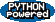EditText of this page (last modified 2010-08-17 19:59:36) FindPage by browsing, title search , text search or an index Or try one of these actions: AttachFile, DeletePage, LikePages, LocalSiteMap, SpellCheck